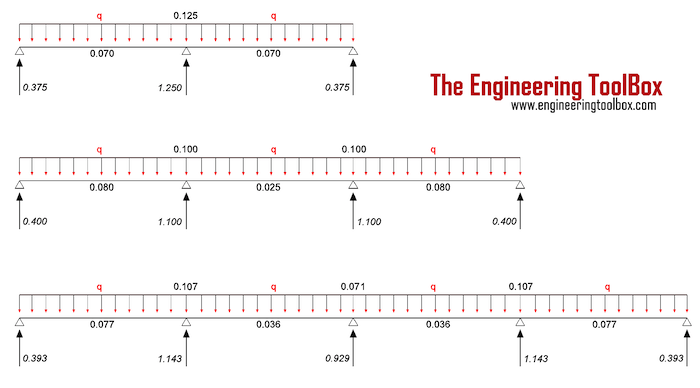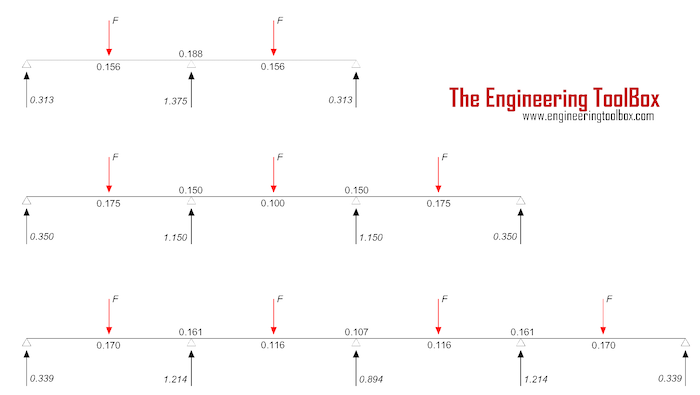Engineering ToolBox - Resources, Tools and Basic Information for Engineering and Design of Technical Applications!

# Continuous Beams - Moment and Reaction Support Forces

## Moments and reaction support forces with distributed or point loads.

### Continuous Beam with Distributed LoadFor a continuous beam with 3, 4 or 5 supports and distributed load the reaction support forces can be calculated as

R = cr q L                     (1)

where

R = reaction support force (N, lbf)

cr = reaction support force coefficient from the figure above

q = distributed load (N/m, lbf/ft)

L = span length (m, ft)

The moments can be calculated as

M = cm q L2                     (2)

where

M = beam moment (Nm, lbf ft)

cm = moment coefficient from the figure above

#### Example - Continuous Beam with Distributed Load

The reaction forces in the end supports for a continuous beam with 3 supports and distributed load 1000 N/m can be calculated as

Rend = (0.375) (1000 N/m)

= 375 N

= 0.38 kN

The reaction force in the center support can be calculated as

Rcenter = (1.250) (1000 N/m)

= 1250 N

= 1.25 kN

The beam moments at the middle of spans with span length 1m can be calculated as

Mend = (0.070) (1000 N/m) (1 m)2

= 70 Nm

The beam moment at the center support can be calculated as

Mcenter = (0.125) (1000 N/m) (1 m)2

= 125 Nm

### Continuous Beam with Point LoadsFor a continuous beam with 3, 4 or 5 supports and point loads the reaction support forces can be calculated as

R = cr F                      (3)

where

cr = reaction support force coefficient from the figure above

F = point load (N, lbf)

The moments can be calculated as

M = cm F L                     (4)

where

cm = moment coefficient from the figure above

#### Example - Continuous Beam with Point Loads

The reaction forces in the end supports for a continuous beam with 3 supports and 2 point loads 1000 N can be calculated as

Rend = (0.313) (1000 N)

= 313 N

= 0.31 kN

The reaction force in the center support can be calculated as

Rcenter = (1.375) (1000 N)

= 1375 N

= 1.4 kN

The beam moments at point loads with span length 1m can be calculated as

Mend = (0.156) (1000 N) (1 m)

= 156 Nm

The beam moment at the center support can be calculated as

Mcenter = (0.188) (1000 N) (1 m)

= 188 Nm

## Related Topics

• Beams and Columns - Deflection and stress, moment of inertia, section modulus and technical information of beams and columns.

## Engineering ToolBox - SketchUp Extension - Online 3D modeling!

Add standard and customized parametric components - like flange beams, lumbers, piping, stairs and more - to your Sketchup model with the Engineering ToolBox - SketchUp Extension - enabled for use with the amazing, fun and free SketchUp Make and SketchUp Pro .Add the Engineering ToolBox extension to your SketchUp from the SketchUp Pro Sketchup Extension Warehouse!

Translate

## Privacy

We don't collect information from our users. Only emails and answers are saved in our archive. Cookies are only used in the browser to improve user experience.

Some of our calculators and applications let you save application data to your local computer. These applications will - due to browser restrictions - send data between your browser and our server. We don't save this data.

## Citation

• Engineering ToolBox, (2017). Continuous Beams - Moment and Reaction Support Forces . [online] Available at: https://www.engineeringtoolbox.com/continuous-beam-moment-reaction-support-forces-distributed-point-loads-d_1988.html [Accessed Day Mo. Year].

Modify access date.

. .

#### Scientific Online Calculator6 24